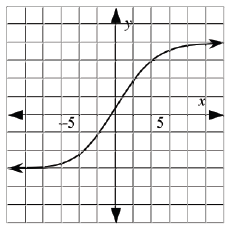### Home > APCALC > Chapter 3 > Lesson 3.3.2 > Problem3-101

3-101.

Draw the following function, given its slope statement below.

The slope starts close to zero. When $x = −5$, the slope increases quickly. Then at $x = 0$ the slope decreases quickly until $x = 5$ when the slope is close to zero again.

Careful! This is a slope statement, not a description of $y$-values.